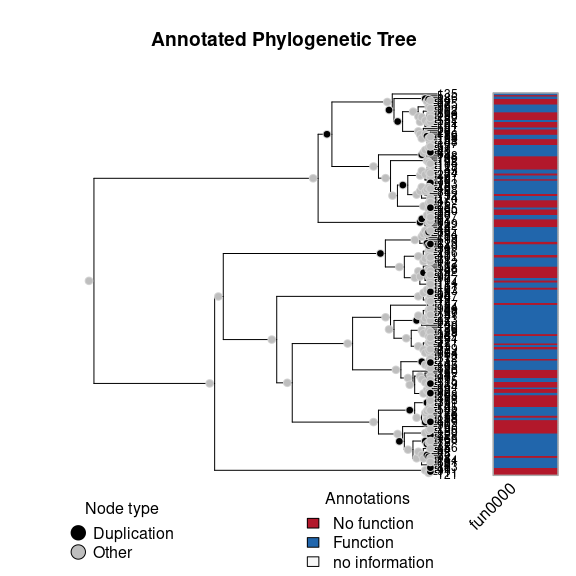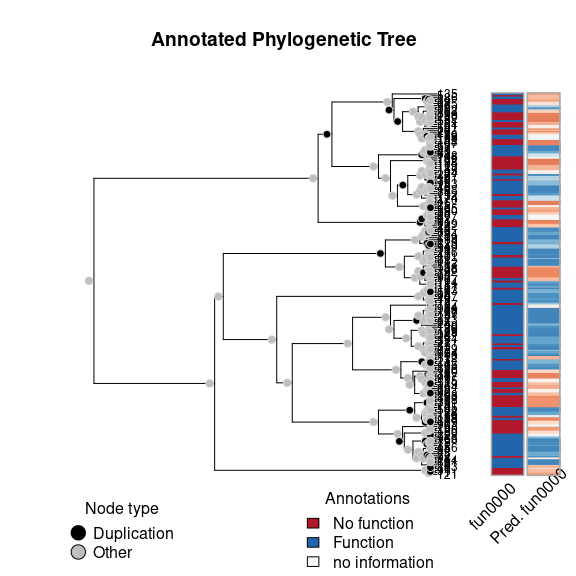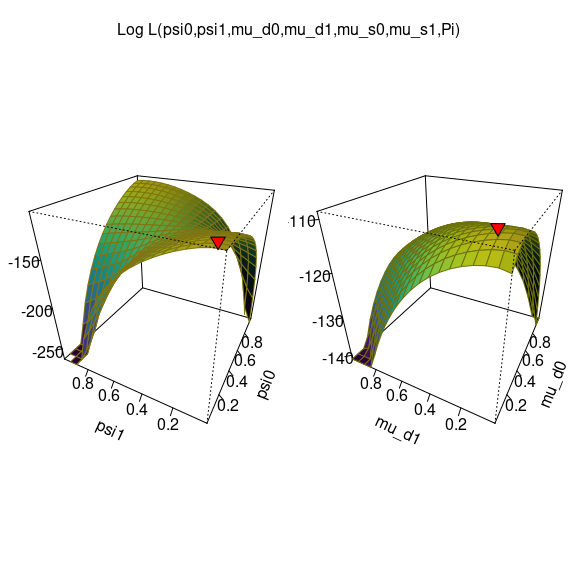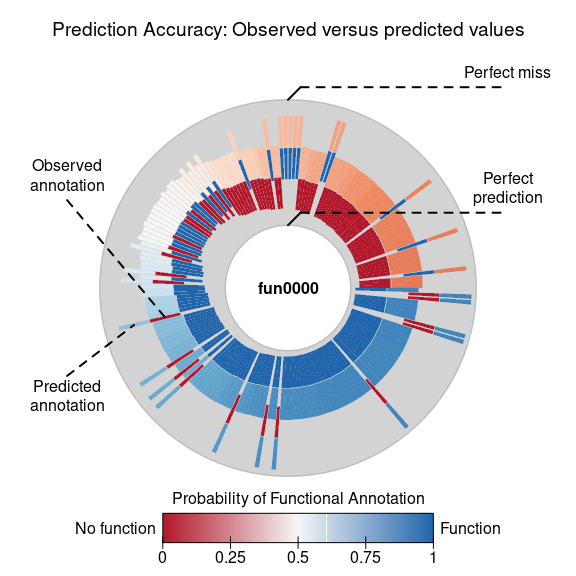# Simulation and Inference

#### August 18, 2021

This is a simple example of simulation, inference, and prediction with the aphylo R package.

## Setup

library(aphylo)
## Loading required package: ape
# Parameter estimates
psi  <- c(.05, .025)
mu_d <- c(.2, .1)
mu_s <- c(.1, .05)
Pi   <- .5

## Simulation

set.seed(223)
x <- raphylo(n = 200, psi = psi, mu_d = mu_d, mu_s = mu_s, Pi = Pi)
plot(x)The simulation function generates an aphylo class object which is simply a wrapper containing:

• a 0/1 integer matrix (annotations),
• a phylo tree (from the ape package), and
• some information about the tree and annotations.

If needed, we can export the data as follows:

# Edgelist describing parent->offspring relations
write.csv(x\$tree, file = "tree.tree", row.names = FALSE)

# Tip annotations
ann <- with(x, rbind(tip.annotation, node.annotation))
write.csv(ann, file = "annotations.csv", row.names = FALSE)

# Event types
events <- with(x, cbind(c(tip.type*NA, node.type)))
rownames(events) <- 1:nrow(events)
write.csv(events, file = "events.csv", row.names = FALSE)

## Inference

To fit the data, we can use MCMC as follows:

ans <- aphylo_mcmc(x ~ psi + mu_d + mu_s + Pi)
## Warning: While using multiple chains, a single initial point has been passed
## via initial: c(0.1, 0.05, 0.9, 0.5, 0.1, 0.05, 0.5). The values will be
## recycled. Ideally you would want to start each chain from different locations.
## Convergence has been reached with 10000 steps. Gelman-Rubin's R: 1.0291. (500 final count of samples).
ans
##
## ESTIMATION OF ANNOTATED PHYLOGENETIC TREE
##
##  Call: aphylo_mcmc(model = x ~ psi + mu_d + mu_s + Pi)
##  LogLik: -109.1906
##  Method used: mcmc (10000 steps)
##  # of Leafs: 200
##  # of Functions 1
##  # of Trees: 1
##
##          Estimate  Std. Err.
##  psi0    0.0792    0.0606
##  psi1    0.0582    0.0396
##  mu_d0   0.2310    0.1396
##  mu_d1   0.1445    0.1062
##  mu_s0   0.1273    0.0429
##  mu_s1   0.0643    0.0324
##  Pi      0.3636    0.2564

For goodness-of-fit analysis, we have a couple of tools. We can compare the predicted values with the observed values:

plot(ans)We can also take a look at the surface of the posterior function

plot_logLik(ans)And we can also take a look at the prediction scores

ps <- prediction_score(ans)
ps       # Printing
## Prediction score (H0: Observed = Random)
##
##  N obs.      : 399
##
##  Observed    : 0.71 ***
##  Random      : NA
##  P(<t)       : 0.0000
## --------------------------------------------------------------------------------
## Values scaled to range between 0 and 1, 1 being best.
##
## Significance levels: *** p < .01, ** p < .05, * p < .10
## AUC 0.85.
## MAE 0.29.
plot(ps) # and plotting